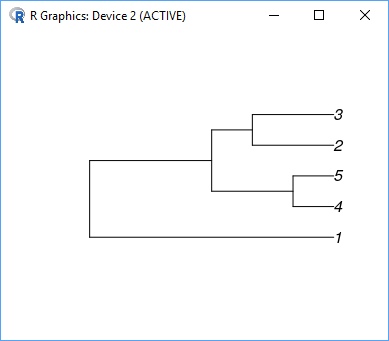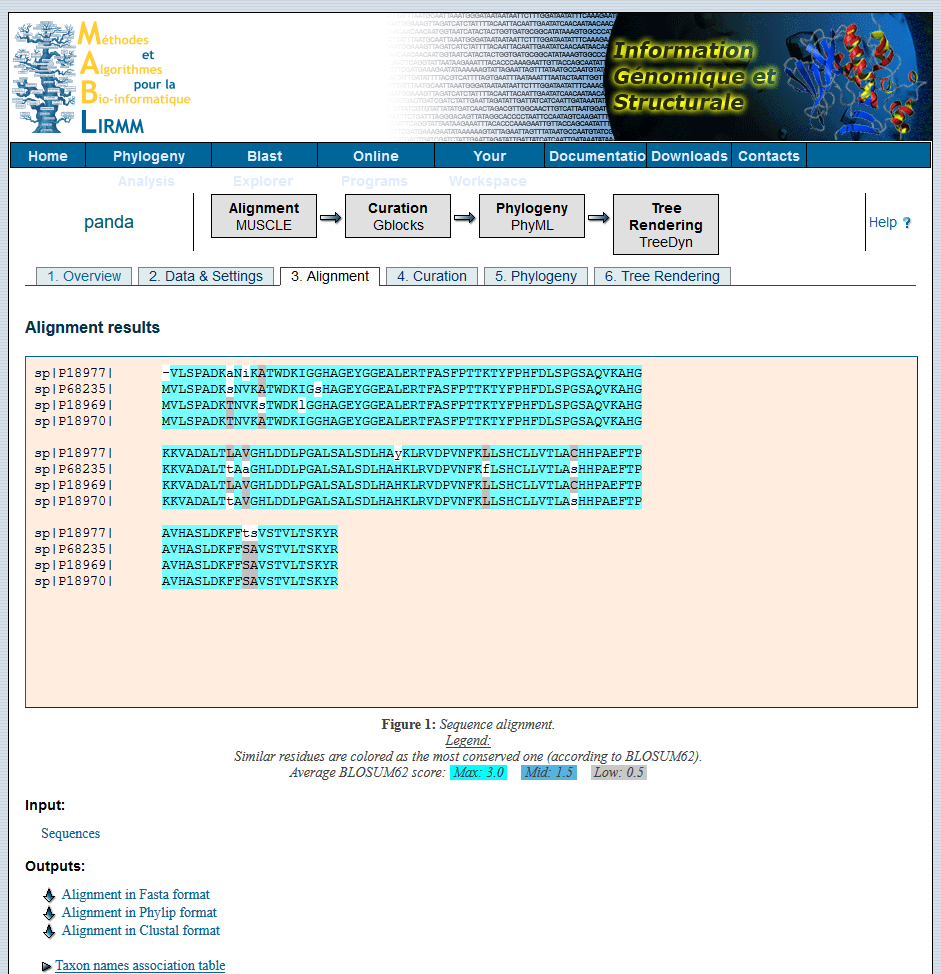# Tutorial 5 - UPGMA, Neighbor Joining

## Problem 1 - UPGMA

Using UPGMA reconstruct the phylogenetic tree that is consistent with the given data.
Assume a set of sequences $A = \{a, b, c, d, e\}$ and the following distance matrix $\mathbf{D}$.

What are ways to calculate $\mathbf{D}$?

$$\mathbf{D} = \begin{bmatrix} 0 & - & - & - & - \\ 12 & 0 & - & - & - \\ 12 & 4 & 0 & - & - \\ 12 & 6 & 6 & 0 & - \\ 12 & 6 & 6 & 2 & 0 \\ \end{bmatrix} \begin{array} \\ a \\ b \\ c \\ d \\ e \end{array}$$

1. Check that the matrix is ultrametric. What is the explanation behind ultrametricity? How can you validate that $\mathbf{D}$ is ultrametric.
2. Reconstruct the phylogenetic tree using the UPGMA algorithm.

## Problem 2 - Neighbor-joining

Using the Neighbor-joining algorithm reconstruct the phylogenetic tree that is consistent with the given data.
In this case, assume a set of sequences $A = \{a, b, c, d\}$ and the corresponding distance matrix $\mathbf{D}$.

$$\mathbf{D} = \begin{bmatrix} 0 & - & - & - \\ 4 & 0 & - & - \\ 10 & 8 & 0 & - \\ 9 & 7 & 9 & 0 \end{bmatrix} \begin{array} \\ a \\ b \\ c \\ d \end{array}$$

Similarly to the previous problem:

1. Before using the neighbor-joining algorithm, check that there exists an additive tree for matrix $\mathbf{D}$. How do you validate that a matrix is additive?
2. If $\mathbf{D}$ is additive, calculate a phylogenetic tree using the neighbor-joining algorithm.

## Problem 3 - Check your answers in the R programming language.

Use the following R code to validate your answers. First, you need to install the following libraries.

R

install.packages("phangorn")
library(phangorn)

Next, you can use the code below to calculate the UPGMA tree …

R

distance <- matrix(c(0,12,12,12,12,
12,0,4,6,6,
12,4,0,6,6,
12,6,6,0,2,
12,6,6,2,0), byrow = TRUE, ncol = 5)
UPGMA <- upgma(as.dist(distance))
plot(UPGMA)
… and the neighbor-joining tree.

R

distance <- matrix(c(0,4,10,9,
4,0,8,7,
10,8,0,9,
9,7,9,0), byrow = TRUE, ncol = 4)
NJ <- NJ(as.dist(distance))
plot(NJ)

The result of the first snippet is below:## Problem 4 - Is Giant panda a bear or a raccoon?

The giant panda was discovered in 1870. Since then the scientists were not able to agree on the classification of this black and white animal living in China. Pandas have several behavioral features that place them in the same taxonomical group as raccoons and red pandas. However visually they remind a bear. We will try to solve this question in the following tutorial.

Initially, biologists had only morphological and behavioral features to build the distance matrix. Use the following table to build the distance matrix.

 Animal Herbivore Tail Bones Hibernation Sound Size Polar bear No Short or none as bear Yes roar big Brown bear No Short or none as bear Yes roar big Giant panda Yes Short or none as raccoon No bleat big Raccoon No Long as raccoon No bleat small Red panda Yes Long as raccoon No bleat small

Once you have the distance matrix, use it to calculate the UPGMA tree or the neighbor-joining tree. Check yourself that the matrix is neither additive nor ultrametric. However, biologists usually use the algorithms anyway as a heuristic. Use the code in R above to calculate the tree.

Can you decide whether the giant panda is raccoon or bear?

To finally solve the issue we should use the standard approach based on sequence similarity. We will use the Hemoglobin alpha protein (HBA for short). In the UniProt database, you can find the HBA sequence for the polar bear, giant panda, raccoon, and red panda. Brown bear HBA is missing; however, there are no doubts about the taxonomy of the brown bear. Hence, we can exclude it from our experiment.

Fill the following file with the HBA protein of giant panda from the UniProt database.

FASTA

>sp|P68235|HBA_URSMA Hemoglobin subunit alpha OS=Ursus maritimus OX=29073 GN=HBA PE=1 SV=2
AVHASLDKFFSAVSTVLTSKYR
>sp|P18977|HBA_PROLO Hemoglobin subunit alpha OS=Procyon lotor OX=9654 GN=HBA PE=1 SV=1
VHASLDKFFTSVSTVLTSKYR
>sp|P18969|HBA_AILFU Hemoglobin subunit alpha OS=Ailurus fulgens OX=9649 GN=HBA PE=1 SV=2
AVHASLDKFFSAVSTVLTSKYR

Ursus maritimus is polar bear in latin, Ailuropoda melanoleuca is giant panda, Procyon lotor is raccoon, and Ailurus fulgens is red panda

Now use the following the online tool on http://www.phylogeny.fr/advanced.cgi to build a phylogenetic tree based on multiple sequence alignment. Is panda a bear or raccoon?The phylogenetic tree is based on multiple sequence alignment. On the contrary, multiple sequence alignment can be calculated from a phylogenetic tree (recall the CLUSTALW algorithm). Use the tree to calculate a new multiple sequence alignment, and use that one to build a new phylogenetic tree. Phylip package contains all the tools needed.

An alternative online tool for phylogeny may be found ENA website. Start with multiple sequence alignment on https://www.ebi.ac.uk/Tools/msa/clustalo/, and finish with phylogeny tool on https://www.ebi.ac.uk/Tools/phylogeny/simple_phylogeny/.

[ Story of panda's taxonomy is mentioned in this book. ]